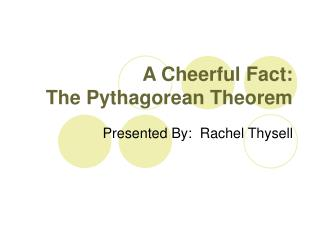DownloadDownload PresentationA Cheerful Fact: The Pythagorean Theorem

# A Cheerful Fact: The Pythagorean Theorem

Télécharger la présentation## A Cheerful Fact: The Pythagorean Theorem

- - - - - - - - - - - - - - - - - - - - - - - - - - - E N D - - - - - - - - - - - - - - - - - - - - - - - - - - -
##### Presentation Transcript

1. A Cheerful Fact: The Pythagorean Theorem Presented By: Rachel Thysell

2. a2 + b2 = c2 • Commonly known that a and b stand for the lengths of the shorter sides of a right triangle, and c is the length of the longest side, or hypotenuse

3. Where did it come from? • Often associated with Pythagoras • Lived 5th Century B.C. • Founder of the Pythagorean Brotherhood • Group for learning and contemplation • However, most commonly heard from authors who wrote many centuries after the time of Pythagoras

4. Where did it come from? • Found in ancient Mesopotamia, Egypt, India, China, and even Greece • Known in China as “Gougo Theorem” • Oldest references are from India, in the Sulbasutras, dating from sometime the first millenium B.C. • The diagonal of a rectangle “produces as much as is produced individually by the two sides.”

5. Famous Triples • All the cultures contained “triples” of whole numbers that work as sides • (3,4,5) is the most famous • a2+b2 = 9+16 = 25 =c2

6. It wasn’t Pythagoras? • A common discovery • Happened during prehistoric times • Theorem came “naturally” • Independently discovered by multiple cultures • Supported by Paulus Gerdes, cultural historian of mathematics • Carefully considered patterns and decorations used by African artisans, and found that the theorem can be found in a fairly natural way

7. Proofs of Pythagorean Theorem • Whole books devoted to ways of proving the Pythagorean Theorem • Many proofs found by amateur mathematicians • U.S. President James Garfield • He once said his mind was “unusually clear and vigorous” when studying mathematics

8. “Square in a Square” • Earliest proof, based on Chinese source • Arrange four identical triangles around a square whose side is their hypotenuse • Since all four triangles are identical, the inner quadrilateral is a square

9. “Square in a Square” • Big square has side a+b, so area is equal to (a+b)2= a2+b2+2ab • Inner square has area c2, and four triangles each with area of ½ab • Big square also equals c2+2ab • Setting them equal to each other, a2+b2+2ab = c2+2ab • Therefore, a2 + b2 = c2

10. Proof using Similar Triangles • Most recent proof • Triangles ACH and CBH are similar to ABC because they both have right angles and share a similar angle • This can be written as AC2=ABxAH and CB2=ABxHB • Summing these two equations, AC2+CB2=ABxAH+ABxHB=ABx(AH+HB)=AB2 • Therefore, AC2+BC2=AB2

11. Euclid’s Elements • Most famous proof of Pythagorean Theorem • 47th Proposition states: “in right-angled triangles the square on the side opposite the right angle equals the sum of the squares on the sides containing the right triangle” • Uses areas, not lengths of the sides to prove. • Early Greek Mathematicians did not usually use numbers to describe magnitudes

12. Euclid’s Proof • The idea is to prove that the little square (in blue) has the same area as the little rectangle (also in blue) and etc. • He does so using basic facts about triangles, parallelograms, and angles.

13. Euclid continues Theorem • There is nothing special about “squares” in the theorem • It works for any geometric figure with its base equal to one of the sides • Their areas equal ka2, kb2, and kc2 • Therefore kc2=k(a2+b2)=ka2+kb2

14. Distance Formula • Also gave birth to the distance formula • Makes classical coordinate geometry “Euclidean” • If distance were measured some other way it would not be Euclidean geometry

15. a2 + b2 = c2 • Pythagorean Theorem remains one of most important theorems • One of most useful results in elementary geometry, both theoretically and in practice

16. The End Any Questions? Thank You!